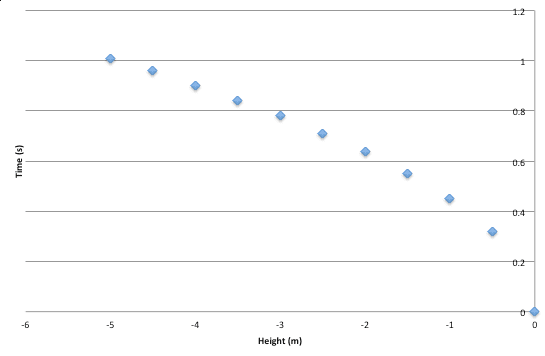# Position-Time Graph to Velocity-Time Graph

• in31l

## Homework Statement

Hi everybody. I just started grade 11 physics, and I'm having some serious trouble translating P-T graphs to V-T graphs. I really just can't seem to grasp the concept of this. It also doesn't help that I'm doing an online course and we're not being taught anything. The experiment has a ball being dropped from 10 different heights, and the effect of how long it takes the ball to reach the ground. I have to turn this graph into a V-T graph. Here it is:The height is on the x-axis because it is controlled. The time is what is being measured. I've tried to convert it, but I really can't grasp the concept of it. Can anyone help? Thanks.

The v-t graph is the slope of the x-t graph.
(do not use "p" for position, p=momentum)

But, to start with, the graph you have is not a position-time graph.
A x-t graph would show the position of the ball at different times.
Yours shows how long the ball takes to fall from different positions - a totally different concept.

It may be useful to work out the final velocity of the ball for different initial heights though I don't know why.
Can you provide the exact problem statement?

Note: in physics the convention about putting the controlled variable on the horizontal axis is not always followed. All you have to do is plot the points in whatever way makes the maths easy. So you can plot heights on the vertical (y) axis if you want to. (Um: why are the heights negative?)

The v-t graph is the slope of the x-t graph.
(do not use "p" for position, p=momentum)

But, to start with, the graph you have is not a position-time graph.
A x-t graph would show the position of the ball at different times.
Yours shows how long the ball takes to fall from different positions - a totally different concept.

It may be useful to work out the final velocity of the ball for different initial heights though I don't know why.
Can you provide the exact problem statement?

Note: in physics the convention about putting the controlled variable on the horizontal axis is not always followed. All you have to do is plot the points in whatever way makes the maths easy. So you can plot heights on the vertical (y) axis if you want to. (Um: why are the heights negative?)

I know, it doesn't really make any sense. Before you start the experiment, there's a question that asks, "Hypothesize what a graph would look like if you were to drop a ball from a certain height". So I roughly made a graph. But then, the actual experiment requires you to drop a ball from 10 different heights, plot it on a graph, then turn that graph into a velocity-time graph. Then it says to compare the real graph with your hypothesized graph. But this doesn't make any sense. Because the hypothesized graph is illustrating what a SINGULAR ball being dropped from a certain height would look like. The real graph is just showing how long it takes for 10 different balls, dropped at different heights, would look like. So they're two different things. But that's what the question is asking, unfortunately. I don't know why we are comparing graphs that are showing two completely different things.

Basically, the height you dropped the ball from will also be how far a ball would have fallen in that time.
This means you can treat it as a position-time graph for a freely falling ball.
Your first task is to plot the time on the horizontal and the height on the vertical.
It should look like a parabola - and so should your initial sketch.

The next step is to find the slope of the tangent to the curve for each point.
You know how to find the slope between each pair of points right?

Basically, the height you dropped the ball from will also be how far a ball would have fallen in that time.
This means you can treat it as a position-time graph for a freely falling ball.
Your first task is to plot the time on the horizontal and the height on the vertical.
It should look like a parabola - and so should your initial sketch.

The next step is to find the slope of the tangent to the curve for each point.
You know how to find the slope between each pair of points right?

But shouldn't the height be on the x-axis and the time on the y-axis, since height is my independent variable, and time is what I'm supposed to find?

A position vs time graph has position (height in this case) on the y-axis and time on the x axis.
So plot it that way. There is nothing wrong with that.

Children get taught that a graphs axes have to cross at point (0,0) and that the dependent variable goes on a specific axis. These are just conventions, not laws of Nature, you don't have to follow them. In science they are usually unhelpful. In physics we just ignore them. We draw our graphs whichever way makes the maths easiest.

•1 person
A position vs time graph has position (height in this case) on the y-axis and time on the x axis.
So plot it that way. There is nothing wrong with that.

Children get taught that a graphs axes have to cross at point (0,0) and that the dependent variable goes on a specific axis. These are just conventions, not laws of Nature, you don't have to follow them. In science they are usually unhelpful. In physics we just ignore them. We draw our graphs whichever way makes the maths easiest.

Thank you. That makes this much easier. I understand how to do it now.